# Algebra II : Circle Functions

## Example Questions

1 2 3 5 Next →

### Example Question #19 : Center And Radius Of Circle Functions

Find the center and radius of the circle given the following equation: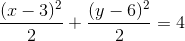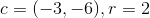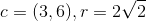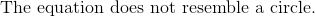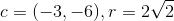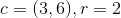Explanation:

The equation of a circle is in the format: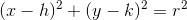where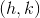is the center andis the radius.

Multiply two on both sides of the equation.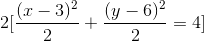The equation becomes: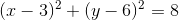The center is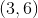.

The radius is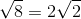.

The answer is:### Example Question #20 : Center And Radius Of Circle Functions

Determine the radius of the circle given by the following function: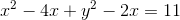Explanation:

To rewrite the given function as the equation of a circle in standard form, we must complete the square for x and y. This method requires us to use the following general form: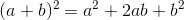To start, we can complete the square for the x terms. We must halve the coefficient of x, square it, and add it to the first two terms: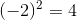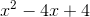Now, we can rewrite this as a perfect square, but because we added 4, we must subtract 4 as to not change the original function: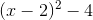We do the same procedure for the y terms: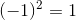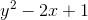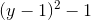Rewriting our function, we get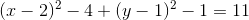Moving the constants to the right side, we get the function of a circle in standard form: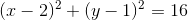Comparing towe see that the radius of the circle is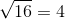Notice that the radius is a distance and can therefore never be negative.

### Example Question #21 : Center And Radius Of Circle Functions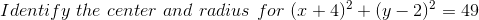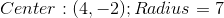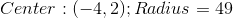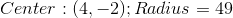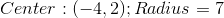Explanation:

When identifying the center of a circle, take the opposite sign of each value connected to x and y.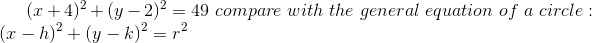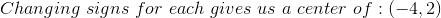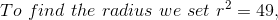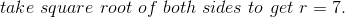### Example Question #22 : Center And Radius Of Circle Functions

What is the center and radius of the following equation, respectively?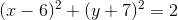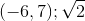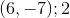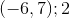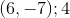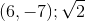Explanation:

The equation given represents a circle.represents the center, andis the radius.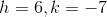The center is at: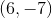Set up an equation to solve the radius.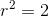The radius is: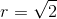The answer is:### Example Question #23 : Center And Radius Of Circle Functions

Which of the following represents the formula of a circle with a radius ofcentered at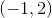?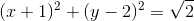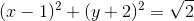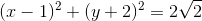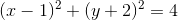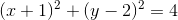Explanation:

Write the standard form for a circle.The circle is centered at:The radius is: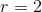Substitute all the known values into the formula.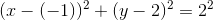Simplify this equation.

The answer is:1 2 3 5 Next →

### All Algebra II Resources